Courses

# Classification of Soils and Clay Minerals Civil Engineering (CE) Notes | EduRev

## Topic wise GATE Past Year Papers for Civil Engineering

Created by: Gate Gurus

## Civil Engineering (CE) : Classification of Soils and Clay Minerals Civil Engineering (CE) Notes | EduRev

The document Classification of Soils and Clay Minerals Civil Engineering (CE) Notes | EduRev is a part of the Civil Engineering (CE) Course Topic wise GATE Past Year Papers for Civil Engineering.
All you need of Civil Engineering (CE) at this link: Civil Engineering (CE)

Q.1 A fine grained soil is found to be plastic in the water content range of 26-48%. As per Indian Standard Classification System, the soil is classified as    [2016 : 1 Mark, Set-I]
(a) CL
(b) CH
(c) CL-ML
(d) CI
Ans.
(D)
Solution:
Soil is plastic in the range of 26-48%.
Hence plastic limit = 26% and liquid limit = 48%. Because liquid limit is in the range of 35% to 50%. Hence intermediate compressible soil.

Q.2 The clay mineral primarily governing the swelling behavior of Black Cotton soil is    [2014 : 1 Mark, Set-II]
(a) Halloysite
(b) lllite
(c) Kaolinite
(d) Montmorillonite
Ans.
(D)

Q.3 As per Indian Standard Soil Classification System (IS 1498 : 1970), an expression for A-line is    [2014 : 1 Mark, Set-II]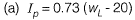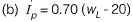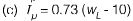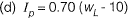Ans.
(A)

Q.4 As per the Indian Standard soil classification system, a sample of silty clay with liquid limit of 40% and plasticity index of 28% is classified as    [2012 : 1 Mark]
(a) CH
(b) CI
(c) CL
(d) CL-ML
Ans.
(B)
Solution: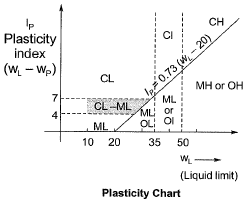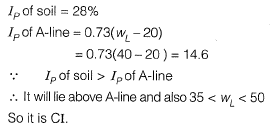Q.5 The results for sieve analysis carried out for three types of sand, P, Q and R, are given in the adjoining figure. If the fineness modulus values of the three sands are given as FMP, FMand FMR, it can be stated that    [2011 : 1 Mark]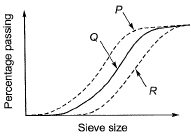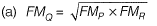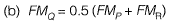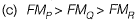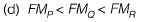Ans.
(A)
Solution:
The term fineness modulus is used to indicate an index number which roughly proportional to average size of particle in entire quantity of aggregate.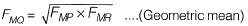Q.6 A fine grained soil has liquid limit of 60 and plastic limit of 20. As per the plasticity chart, according to IS classification, the soil is represented by the letter symbols    [2010 : 1 Mark]
(a) CL
(b) CI
(c) CH
(d) CL-ML
Ans.
(C)
Solution:
Plasticity index,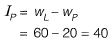Equation of A line is given by,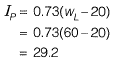Thus the given soil lies above the A-Line. The liquid limit of the soil is more than 50, hence the soil will be CH.

Offer running on EduRev: Apply code STAYHOME200 to get INR 200 off on our premium plan EduRev Infinity!

69 docs

,

,

,

,

,

,

,

,

,

,

,

,

,

,

,

,

,

,

,

,

,

;